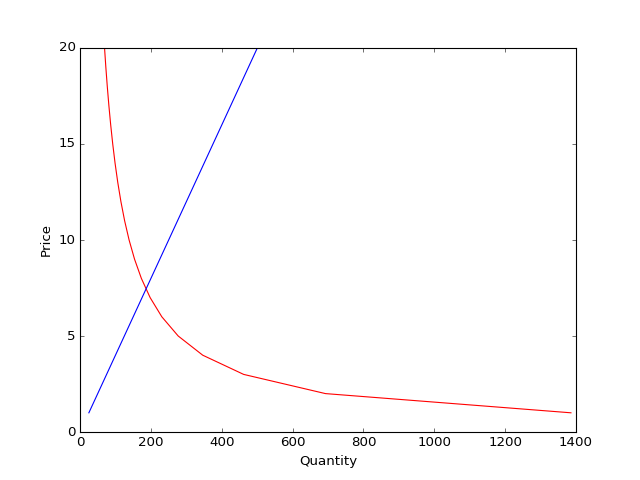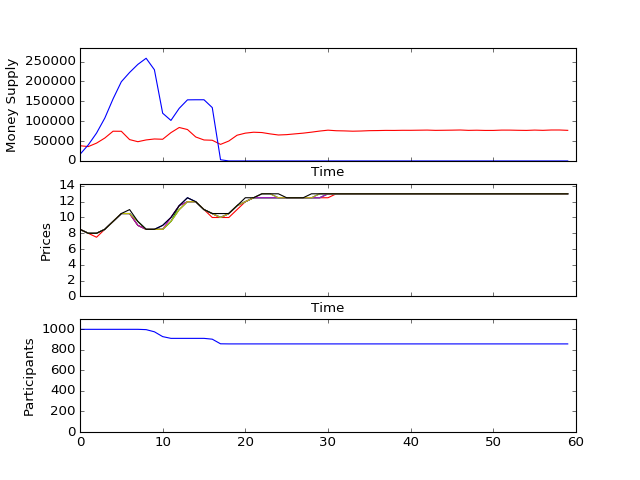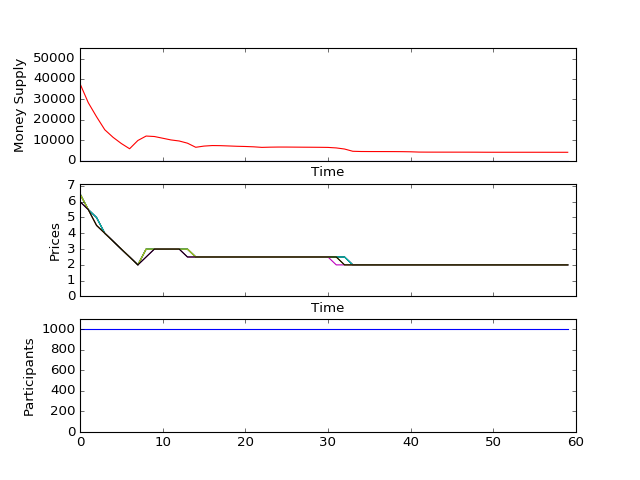In :
%matplotlib notebook

In :
import random
import math
import numpy as np
import matplotlib.pyplot as plt
import time
from sklearn.utils.extmath import cartesian


Hello there, If you have found this through reddit or social media sharing sites, you should be aware that this iPython Notebook is a companion piece to a talk I gave at Sydney Python. The full write-up can be found here and the slides that precede and succeed this simulation can be found here. I recommend reading in this order:

1. Slides (until Let's Simulate)
2. This simulation
3. Slides (for some takeaway bullet points)
4. Blog post for further discussions

Also, come caveats: I have purposely omitted discussions of stuff such as the effect of money supply and demand on wealth. It should be quite immediately obvious the moment you start thinking of money as a good as well. However, I have found through previous experience of teaching this to people, that it's not a good way to help them understand how money is created

Welcome to the great nation of Dystopianistan. The eternal leader is Kimberly Il-Smith. Here in Dystopianistan, things are done slightly differently from how you know them to be. But there are some quite familiar things. We'll start with one of them: the idea of Banks

Banks provide a place for the citizens of Dystopianistan to store their money. It also provides loans to any citizen who needs it. Loans in Dystopianistan are rather simple - there is no concept of compound interest. Also, all loans are set for a fixed period of time

Banks loan money with special rules - they can loan more than they actually have. If a Bank holds \$200 in its holdings, it can loan up to \$1000. The reason is because through a lot of study, it's been found that the citizens of Dystopianistan are generally good debtors - they pay back whatever they owe, plus interest, most of the time. This allows the banks to count on future returns, and so they're allowed to lend out more money than they have in store.

In :
interest_rate = 0.1
reserve_rate = 0.2

class Bank(object):
def __init__(self, interest_rate, reserve_rate):
self.interest_rate = interest_rate
self.reserve_rate = reserve_rate
self.loans = []
self.unrecovered = 0.0
self.holding = 0.0
def can_lend(self, amount):
# check if there is enough money in holdings
if self.unrecovered+amount > 1/self.reserve_rate*self.holding:
return False # cannot loan any more money
return True
def lend(self, loan):
self.unrecovered += loan.amount
def repay(self, loan):
self.unrecovered -= loan.repay_amount()

class Loan(object):
def __init__(self, lender, borrower, amount, repay_at, interest_rate):
self.lender = lender
self.borrower = borrower
self.amount = amount
self.repay_at = repay_at
self.interest_rate = interest_rate
def repay_amount(self):
return self.amount * (1 + self.interest_rate)
def __repr__(self):
return "{} | {}".format(self.repay_amount(), self.repay_at)

central_bank = Bank(interest_rate, reserve_rate)


In Dystopianistan, citizens are also able to make investments. Investments are also simple here. At the end of 7 periods, citizens get a return on investment by means of upgrading their skills - they simply become more productive depending on how much investment they put in.

In :
class Investment(object):
def __init__(self, principle, return_rate, return_at):
self.principle = principle
self.return_rate = return_rate
self.return_at = return_at
self.p = abs(random.gauss(0, 0.01)) # half normal distribution
def __repr__(self):
return "{} | {}".format(self.principle, self.return_at)


The citizens of Dystopianistan are very different from what you normally know as people. They are born as full adults, with the capacity to produce things. Right from the moment they are born, they are thrust into the economic machine, producing and consuming goods. Due to their highly efficient nature, the citizens of Dystopianistan only consume goods once every period. Likewise, they produce goods only once every period.

Despite all that, the citizens of Dystopianistan are not robots. They are quite varied. Each citizen has different likes and dislikes (we call those preferences), and each citizen has different skills in producing goods (we call those productivities). Also, each citizen of Dystopianistan have different preferences for risk - some like to save more money now (savings_pref), some like to invest more money for future benefit (investment_pref).

Consumption of goods make Dystopianistanians happy - a citizen may be good at producing oranges, but she may like apples more than oranges. Therefore she does the logical thing - sell her oranges and buy apples instead. However, sometimes, the prices of apples may be too high for her consumption habit, so she has to either cut down her consumption of apples, or take out a loan.

Consequently, every Dystopianistanian also has a likelihood of taking out a loan to fuel his/her spending habits (we call that consumerism).

In determining if a citizen is qualified for a loan, we'd have to calculate their creditworthiness.

In :
DEFAULT_THRESHOLD = 4
class Person(object):
def __init__(self, bank, endowment, productivities, preferences, investment_pref, savings_pref):
'''
:param bank: which Bank does this Person banks with?
:param endowment: how much savings does a person initially start with? rich families = high endowment
:param productivities: a list of numbers the size of basket.
This represents how many goods Person can produce per period.
:param preferences: a list of floats the size of basket
:param investment_pref: a float between [0,1). 0 = person doesn't care about his/her future earnings
'''
self.bank = bank
self.savings = endowment
self.bank.holding += self.savings
self.productivities = productivities
self.preferences = preferences
self.investment_pref = investment_pref
self.savings_pref = savings_pref
self.loans = []
self.investments = []
self.last_income = endowment
self.nonpayment = 0
self.defaulted = False
self.consumerism = np.random.beta(3,5)

def utility(self, q, investment):
'''
utility represents a utility a Person has when presented with an allocation of goods and investment.

:param q: a list of quantities
:param investment: a number
'''
u = 1
for g, p in zip(q, self.preferences):
u *= math.pow(g, p)
u *= math.pow(investment, self.investment_pref)
return u

def normalized_preferences(self):
return [pref/(sum(self.preferences)+self.investment_pref+self.savings_pref) for pref in self.preferences]

def normalized_productivities(self):
return [prod/sum(self.productivities) for prod in self.productivities]

def produce(self, priceMatrix, current):
'''
produce simulates the production capactity of a Person.
'''
normal_prod = np.array(self.normalized_productivities())
budget = self.budget(current)
quantities = normal_prod * priceMatrix
return quantities

def consume(self, priceMatrix, current):
'''
Returns the demand given the prices. Demand is inelastick, because these Persons are turdballs.
'''
normal_pref = np.array(self.normalized_preferences())
budget = self.budget(current)
quantities = normal_pref * budget / priceMatrix
return quantities

def budget(self, current):
'''
The budget forms the constraint for a Person in his/her consumption
'''
repayments = 0.0
for loan in self.loans:
if current == loan.repay_at:
repayments += loan.repay_amount()
return self.creditworthiness()*self.consumerism + self.savings - (1-self.consumerism) * repayments

def creditworthiness(self):
'''
creditworthiness determines how much a Person can borrow from the bank
'''
if self.savings > 0:
return self.last_income + (self.last_income * interest_rate) + self.savings
elif self.last_income > 0:
return self.last_income + (self.last_income * interest_rate)
return 0 # NO CREDIT FOR YOU!

def debt(self):
'''
How much debt does a Person have?
'''
amount = 0.0
for loan in self.loans:
amount += loan.repay_amount()
return amount

def spend(self, amount, current):
if amount < self.savings:
self.savings -= amount
return
elif amount > self.savings:
if self.bank.can_lend(amount):
loan = Loan(self.bank, self, self.creditworthiness(), current+5, interest_rate)
self.loans.append(loan)
self.bank.lend(loan)
else:
self.savings = 0

def earn(self, income):
self.savings += income
self.last_income = income

def invest(self, amount, current):
investment = Investment(amount, interest_rate, current+7)
self.investments.append(investment)

def step(self, prices, current):
production = self.produce(prices, current)
income = sum([p*q for p, q in zip(prices, production)])
consumption = self.consume(prices, current)

inv_pref = self.investment_pref/(sum(self.preferences)+self.investment_pref)
inv_amt = inv_pref * self.budget(current)

norm_prod = self.normalized_productivities()
prod_cost = [p*q for p, q in zip(prices, production)]
prod_cost = sum(n*c for n, c in zip(norm_prod, prod_cost))

cost = sum([p*q for p, q in zip(prices, consumption)]) + inv_amt + prod_cost
self.earn(income)
self.spend(cost, current)
if self.savings > inv_amt:
self.invest(inv_amt, current)

# remove loans that are up
remove = []
cantpay = False
for loan in self.loans:
if loan.repay_at <= current:
if self.savings >= loan.repay_amount():
self.savings -= loan.repay_amount()
self.bank.repay(loan)
remove.append(loan)
else:
cantpay = True
for loan in remove:
self.loans.remove(loan)

if cantpay:
self.nonpayment += 1

# realize investments
remove = []
for investment in self.investments:
if investment.return_at == current:
self.savings += investment.principle*(1+investment.return_rate)
self.productivities = [prod *(1+investment.p) for prod in self.productivities]
remove.append(investment)
for investment in remove:
self.investments.remove(investment)

# check for defaults
if self.nonpayment >= DEFAULT_THRESHOLD:
self.defaulted = True
default_amt = 0.0
for loan in self.loans:
default_amt += loan.amount
self.bank.unrecovered -= default_amt


In :
basket = 3
endowment = random.gauss(50,10)
investment_pref = np.random.randint(0,10)
savings_pref = np.random.randint(0,10)
A = Person(central_bank,endowment, skills, preferences, investment_pref, savings_pref)

for i in range(2):
prices = np.random.beta(3,2, (3,basket)) * 10
production = A.produce(prices,i)
income = sum([p*q for p, q in zip(prices, production)])
consumption = A.consume(prices,i)
consumes = sum([p*q for p,q in zip(prices, consumption)])
A.step(prices, i)

print("Step {}".format(i))
print("Price: \n{}".format(prices))
print("Budget {}".format(A.budget(i)))
print("Productivity \n{}".format(production))
print("Consumption \n{}".format(consumption))
print("Income: {} {}".format(A.last_income, income))
print("Savings: {}".format(A.savings))
print("Cost: {}".format(consumes))
print("Debt: {}".format(A.debt()))
print("Creditworthiness {}".format(A.creditworthiness()))
print("Loans: {}".format(A.loans))
print("Investments: {}".format(A.investments))
print("\n\n")

Step 0
Price:
[[ 5.60191322  7.01336885  7.12751418]
[ 8.66811908  4.56525387  7.09286451]
[ 7.99165882  8.03726514  3.87125812]]
Budget 189.01043990690778
Productivity
[[ 2.40081995  0.          4.07286525]
[ 3.71490818  0.          4.05306543]
[ 3.42499664  0.          2.2121475 ]]
Consumption
[[  0.           4.57357645   6.00044237]
[  0.           7.0261544    6.0297554 ]
[  0.           3.99093199  11.04763277]]
Income: 42.47858983516505 [ 73.02185606   0.          66.34104276]
Savings: 97.47168364658936
Cost: [   0.           96.22853572  128.3047143 ]
Debt: 158.61794571179803
Creditworthiness 144.19813246527093
Loans: [158.61794571179803 | 5]
Investments: [16.038089286975342 | 7]

Step 1
Price:
[[ 7.63988269  4.41222354  8.21191414]
[ 8.87513079  4.20549432  2.89029569]
[ 3.12333561  5.97389287  6.57323107]]
Budget 307.61531531020654
Productivity
[[ 3.27423544  0.          4.69252237]
[ 3.80362748  0.          1.65159754]
[ 1.3385724   0.          3.75613204]]
Consumption
[[  0.          10.70947777   7.67220393]
[  0.          11.2359229   21.79828181]
[  0.           7.90985225   9.58485702]]
Income: 63.549365420926776 [ 62.95327685   0.          67.99811986]
Savings: 161.02104906751612
Cost: [   0.          141.75782993  189.01043991]
Debt: 158.61794571179803
Creditworthiness 230.92535103053558
Loans: [158.61794571179803 | 5]
Investments: [16.038089286975342 | 7, 23.626304988363472 | 8]



Consider a small subset of the population of Dystopianistan where there are two citizens, Alice and Bob, and they only produce two goods: apples and oranges.

This is what they produced:

WhoApples ProducedOranges Produced
Alice510
Bob125

This is what each of them prefer to have:

WhoApples PreferedOranges Prefered
Alice82
Bob913

This can be solved quite easily with basic algebra:

$$3A - 8O = 0$$$$A = \frac{8}{3}O$$

While it was simple to solve for Alice and Bob, the process of solving it for everyone take $\mathcal{O}(n^n)$. Instead, the geniuses at Dystopianistan came up with a novel idea:

1. Poll everyone to see what they would produce given that a good is at a certain price.
2. Poll everyone to see what they would consume given that a good is at a certain price.
3. Calculate a fair price for every good in the world
4. Everyone by law must sell all the goods they produce per period and buy whatever they want at that price.
5. They would build a supercomputer to calculate all the prices - it'd be called InvisibleHandatron 2000.

Through meticulous study, they have discovered that this method is indeed the most fair method of redistributing the goods - at least in theory. The end result was Pareto optimal - where no one can be made better off without making someone worse off.

So, back to the example with Alice and Bob. Let's say the fair price for Apples are \$3, and the fair price for oranges are \$8.

First, they both sell all their goods to InvisbleHandatron 2000:

WhoIncome From ApplesIncome from OrangesTotal Income
Alice\$15\$80\$95 Bob\$36\$40$76

They then buy back however much they prefer from InvisibleHandatron 2000:

Alice\$24\$20\$45 Bob\$27\$104\$131

Here you might notice Bob doesn't make quite enough money to buy what he prefers. But who knows? He may crack into his savings. Or he might take a loan to fund his fruit-related addiction. Or he may choose to eat fewer fruits and go to the toilet less. Either way, the story has ended.

In :
class Market(object):
current = 0
def __init__(self, thickness, basket, bank, max_price, steps):
self.participants = []
self.bank = bank
self.max_price = max_price # government intervention! This is the price ceiling that the government sets
self.steps = steps         # how many steps to break down from 0 to max price
for i in range(thickness):
endowment = random.gauss(50,10)
investment_pref = np.random.randint(0, 10)
savings_pref = np.random.randint(0,10)
participant = Person(bank,endowment, skills, preferences, investment_pref, savings_pref)
self.participants.append(participant)
def demand(self, priceMatrix):
quantities = np.zeros((priceMatrix.shape, priceMatrix.shape))
for participant in self.participants:
quantities += participant.consume(priceMatrix, self.current)
return quantities

def supply(self, priceMatrix):
quantities = np.zeros((priceMatrix.shape, priceMatrix.shape))
for participant in self.participants:
q = participant.produce(priceMatrix, self.current)
quantities += q
return quantities

def market_price(self):
prices = (np.arange(self.steps) + 1) * self.max_price / float(self.steps)
#         priceMatrix = cartesian([prices for i in range(m.basket)])

supply = self.supply(priceMatrix)
demand = self.demand(priceMatrix)
surplus = supply - demand
min_surplus = np.argmin(np.abs(surplus),0)
eq_prices = []
eq_prices.append(priceMatrix[min_surplus[i]][i])
return np.array(eq_prices)

def step(self):
eq_prices = self.market_price()
for participant in self.participants:
if not participant.defaulted:
central_bank.holding -= participant.savings
participant.step(eq_prices, m.current)
central_bank.holding += participant.savings

m.current += 1

def m1(self):
money = 0.0
for participant in self.participants:
if not participant.defaulted and participant.savings > 0:
money += participant.savings
return money
def m2(self):
money = 0.0
for participant in self.participants:
if not participant.defaulted:
for loan in participant.loans:
money += loan.amount * (1+loan.interest_rate)
return money

def actives(self):
return sum([1 for p in self.participants if not p.defaulted])


The market is quite self explanatory - at every time period, it calculates the equilibrium price. Remember, the citizens of Dystopianistan are price takers with InvisbleHandatron being the price maker.

Here we see how the price is calculated:

In :
basket = 4
m = Market(100, basket, central_bank, 20, 20)
prices = (np.arange(m.steps) + 1) * m.max_price / float(m.steps)
# priceMatrix = cartesian([prices for i in range(m.basket)])
priceMatrix = np.tile(prices.reshape(m.steps,1), ( 1, basket))
supply = m.supply(priceMatrix)
demand = m.demand(priceMatrix)
market_price = m.market_price()
print("Market Price: {}".format(market_price))

fig, ax = plt.subplots(1,1)
ax.set_xlabel("Quantity")
ax.set_ylabel("Price")
ax.plot(demand.T,priceMatrix.T, c='r')
ax.plot(supply.T,priceMatrix.T, c='b')
fig.canvas.draw()

Market Price: [ 7.  8.  8.  8.]It should come to no surprise that the chart looks very close to the traditional supply-demand charts you see in economics text books. Afterall, the citizens of Dystopianistan are generally quite rational.

In :
def plotmoney(ax, steps, m1s, m2s):
ax.lines.set_xdata(steps)
ax.lines.set_xdata(steps)
ax.lines.set_ydata(m1s)
ax.lines.set_ydata(m2s)

def plotpeople(ax, steps, people):
ax.lines.set_xdata(steps)
ax.lines.set_ydata(people)

def plotprices(ax, steps, goods):
for i, g in enumerate(goods):
ax.lines[i].set_xdata(steps)
ax.lines[i].set_ydata(g)


And now, the simulation of how things work in Dystopianistan. Recall earlier that Dystopianistan has discovrered that the citizens are generally good payers-back of loans? Well, here's a how's-the-sausage-is-made reasoning for that: they simply throuw debtors who have failed to pay back their loans DEFAULT_THRESHOLD times, into the gulag, removing them from the economy entirely.

It would make sense too. If you analyze their behaviour, the defaulters generally have high consumerism preferences. They typicallly spend more than they can make. Therefore in her Great Knowledge, the Eternal Glorious Leader of Dystopianistan has decided to cast those who default into little boats along the river (the Eternal Glorious Leader has plans with those boats obviously - to send them to explore Terra Australis Incognita in about 10 years time)

In :
#reset in case the below case "no loans allowed" is run previously
def budget(self, current):
'''
The budget forms the constraint for a Person in his/her consumption
'''
repayments = 0.0
for loan in self.loans:
if current == loan.repay_at:
repayments += loan.repay_amount()
return self.creditworthiness()*self.consumerism + self.savings  - (1-self.consumerism) * repayments
Person.budget = budget
central_bank = Bank(interest_rate, reserve_rate)

n_steps = 60
participant_count = 1000
max_price = 100
price_steps = 200
m = Market(participant_count, basket, central_bank, max_price, price_steps)
print(central_bank.holding)
print(len(m.participants))
mp = m.market_price()

fig,(ax1,ax2, ax3) = plt.subplots(3,1, sharex=True)
ax1.set_xlabel('Time')
ax1.set_ylabel('Money Supply')
ax1.set_xlim(0,n_steps)
ax1.set_ylim(0,700000)
ax2.set_xlabel('Time')
ax2.set_ylabel('Prices')
ax2.set_xlim(0,n_steps)
ax2.set_ylim(0,35)
ax3.set_ylabel("Participants")
ax3.set_ylim(0,participant_count*1.1)

steps =
m1s = [m.m1()]
m2s = [m.m2()]
participants = [participant_count]
prices = [[p] for p in mp]
colors = ['r', 'b', 'g', 'c', 'm', 'y','k']

ax1.plot(steps, m1s, c='r')
ax1.plot(steps, m2s, c='b')
ax3.plot(steps, participants)
for i, p in enumerate(prices):
ax2.plot(steps, p, c=colors[i])
fig.canvas.draw()

for i in range(n_steps):
mp = m.market_price()
m.step()

steps.append(i)
m1 = m.m1()
m2 = m.m2()

# plotting shit
m1s.append(m1)
m2s.append(m2)
for i, p in enumerate(mp):
prices[i].append(p)

participants.append(m.actives())

maxY1 = max(m1s+m2s)*1.1
maxY2 = max([p for pl in prices for p in pl ])*1.1
ax1.set_ylim(0, maxY1)
ax2.set_ylim(0, maxY2)
plotmoney(ax1, steps, m1s, m2s)
plotprices(ax2, steps, prices)
plotpeople(ax3, steps, participants)

fig.canvas.draw()
#     print("Time {}. M1: {}, M2: {}, Total: {}".format(i, m1, m2, m1+m2))


50361.26007423992
1000What happens if loans cannot be made?

In :
import types
central_bank2 = Bank(interest_rate, reserve_rate)
central_bank2.can_lend = types.MethodType(lambda bank, amount: False, central_bank2) # never do this on prod servers!
Person.budget = lambda self, cuurent: self.savings


As you'll note - nothing interesting happens if there are no loans allowed. The economy stagnates. A stagnating economy usually spells civil unrest

In :
m = Market(participant_count, basket, central_bank2, max_price, price_steps)
print(central_bank2.holding)
print(len(m.participants))
mp = m.market_price()

fig,(ax1,ax2, ax3) = plt.subplots(3,1, sharex=True)
ax1.set_xlabel('Time')
ax1.set_ylabel('Money Supply')
ax1.set_xlim(0,n_steps)
ax1.set_ylim(0,700000)
ax2.set_xlabel('Time')
ax2.set_ylabel('Prices')
ax2.set_xlim(0,n_steps)
ax2.set_ylim(0,35)
ax3.set_ylabel("Participants")
ax3.set_ylim(0,participant_count*1.1)

steps =
m1s = [m.m1()]
m2s = [m.m2()]
participants = [participant_count]
prices = [[p] for p in mp]
colors = ['r', 'b', 'g', 'c', 'm', 'y','k']

ax1.plot(steps, m1s, c='r')
ax1.plot(steps, m2s, c='b')
ax3.plot(steps, participants)
for i, p in enumerate(prices):
ax2.plot(steps, p, c=colors[i])
fig.canvas.draw()

for i in range(n_steps):
mp = m.market_price()
m.step()

steps.append(i)
m1 = m.m1()
m2 = m.m2()

# plotting shit
m1s.append(m1)
m2s.append(m2)
for i, p in enumerate(mp):
prices[i].append(p)

participants.append(m.actives())

maxY1 = max(m1s+m2s)*1.1
maxY2 = max([p for pl in prices for p in pl ])*1.1
ax1.set_ylim(0, maxY1)
ax2.set_ylim(0, maxY2)
plotmoney(ax1, steps, m1s, m2s)
plotprices(ax2, steps, prices)
plotpeople(ax3, steps, participants)

fig.canvas.draw()
#     print("Time {}. M1: {}, M2: {}, Total: {}".format(i, m1, m2, m1+m2))

50120.42960338321
1000